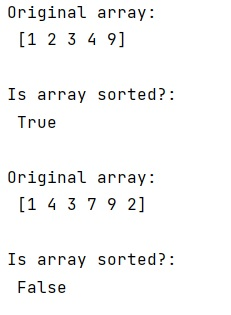# Check if a NumPy array is sorted (in ascending order)

Learn, how to check if a NumPy array is sorted (in ascending order) in Python? By Pranit Sharma Last updated : March 28, 2023

Suppose that we are given a 1D numpy array that contains some integer values and we need to check if all the values of this numpy array are arranged in ascending order or not or simply we need to apply a check for a sorted array.

## Checking if a NumPy array is sorted

To check if a NumPy array is sorted (in ascending order), we can use a comprehension function or a lambda function where we can use numpy.all() where we can pass this condition; arr[:-1] <= arr[1:] and it will return True if all the elements are sorted and False otherwise.

Let us understand with the help of an example,

## Python code to check if a NumPy array is sorted (in ascending order)

```# Import numpy
import numpy as np

# Creating a array
arr = np.array([1,2,3,4,9])

# Display Original array
print("Original array:\n",arr,"\n")

# defining a function
is_sorted = lambda a: np.all(a[:-1] <= a[1:])

# Checking for sorted values
res = is_sorted(arr)

# Display result
print("Is array sorted?:\n",res,"\n")

# Creating a array
arr = np.array([1,4,3,7,9,2])

# Display Original array
print("Original array:\n",arr,"\n")

# Checking for sorted values
res = is_sorted(arr)

# Display result
print("Is array sorted?:\n",res,"\n")
```

### Output